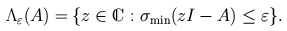Home | Introduction | Software | Examples | History | People | Bibliography | Applications | Theorems

## A Brief Survey of Computational Methods

A catalog of software, including the EigTool for MATLAB, is included further down this page.

Though pseudospectra were defined theoretically as early as 1975, the first published numerical computation appeared in 1987 [Dem87a] - a 3-by-3 matrix. By 1993, extensive computations involving matrices of dimension 64 were being applied to investigate the behavior of differential operators of fluid mechanics [RSH93]. By 2000, examples of dimensions in the hundreds of thousands had been computed [WT01].

Techniques for the computation of pseudospectra have advanced greatly over the past five years. Almost all efforts have been directed towards computing the 2-norm pseudospectra, which can be characterized in terms of the minimum singular value of zI-A,Most algorithms fall into two two broad classes:

• Grid algorithms
Pick a region in the complex plane, cover it with a grid, and compute the minimum singular value of zI-A for each z on the grid.

• Path-following algorithms
Find a point on the boundary of the desired pseudospectrum and follow from it a curve in the complex plane on which the minimum singular value of zI-A is constant. See [BG01], [BG02], [Brü96], [MP00], [MP02].

Grid algorithms, which are the standard methods, face several challenges. One must first choose a region of the complex plane to investigate, and then very many grid points may be necessary to resolve the desired pseudospectral contours adequately. See [BMT97] for a discussion of the first difficulty and [KG02] for a technique to eliminate some of the uninteresting grid points quickly. Another approach to this latter problem was suggested by Gallestey, whose "SH algorithm" uses the sub-harmonicity of the resolvent norm to recursively eliminate portions of the computational domain [Gal98].

Path-following algorithms also have limitations. One must first find a point on the desired contour; if the pseudospectrum is disconnected, each component must be found; and multiple runs are required if one seeks pseudospectra for several different values of epsilon.

Both kinds of algorithm require evaluation of the minimum singular value of zI-A for many different values of z. Considerable progress has been made in making this process as efficient as possible. When A is a dense matrix, Lui noted the advantage of first reducing A to Hessenberg or triangular form before computing the minimum singular value of zI-A [Lui97]. An iterative method can then be used to compute the minimum singular value. Using inverse iteration or inverse Lanczos iteration to compute the minimum singular value seems to be worthwhile for all but the smallest dense matrices, and is essential if A has sparse structure that must be preserved; see also [BH96].

Another speedup technique, which can be applied independently of others, has proved crucial in some applications. When A is large and one only seeks the pseudospectra in a portion of the complex plane, it may be advantageous to project A orthogonally onto a suitably chosen subspace to reduce the problem dimension; see [Lui97], [RSH93], [TT96], [WT01].

For a more detailed tutorial survey of computational techniques for matrices of dimension N<1000, see [Tre99a], where it is claimed that combining various speedups can often accelerate the naive SVD grid algorithm for computing pseudospectra by a factor of about N/4. For larger matrices of dimension up to 106, see [WT01].

The table below lists several different publicly available grid algorithms. At the present, we are unaware of any public domain path-following code for computing pseudospectra. Such software would be a welcome addition.

## Publicly Available Pseudospectra Software

• EigTool, MATLAB routines (formerly the Pseudospectra GUI)
 Author: Tom Wright, Oxford University Description: Graphical user interface, based on fast inverse Lanczos iteration on a grid, with optional projection onto a spatially-localized invariant subspace. Provides a graphical front-end to ARPACK for large-scale computations, followed by efficient pseudospectra approximation. Reference: T. G. Wright and L. N. Trefethen Large-scale computation of pseudospectra using ARPACK and Eigs SIAM J. Sci. Comp. 23 (2001), 591-605. Link to Article

• PPAT, GUI coded in C++
 Author: Dany Mezher, Bernard Philippe, and Wajdi Najem Description: Path following algorithm with X-windows graphical interface, distributed computation. Reference: D. Mezher A graphical tool for driving the parallel computation of pseudospectra Proc. ACM International Conference on Supercomputing, Sorrento, Italy, June 2001, p. 270-276. Link to Article

• `psa.m`, MATLAB script
 Author: Nick Trefethen, Oxford University Description: Inverse-Lanczos iteration on a regular grid Reference: L. N. Trefethen Computation of pseudospectra Acta Numerica (1999), 247-295.

• Grid algorithm and path following codes, MATLAB and Fortran
 Author: Constantine Bekas, University of Patras Description: Software for both the grid algorithm and the path following algorithm. There are MATLAB routines as well as Fortran codes that use ARPACK and SPARSKIT. Reference: See the pseudospectra pages at the University of Patras.

• `pscont.m` and `ps.m`, MATLAB scripts from the Test Matrix Toolbox, Version 3.0
 Author: Nick Higham, University of Manchester Description: `pscont.m` uses MATLAB's full `svd` command on a regular grid. `ps.m` plots eigenvalues of dense random perturbations of the matrix. Reference: N. J. Higham The Test Matrix Toolbox for MATLAB (Version 3.0) University of Manchester Numerical Analysis Report 276, September 1995. download as gzipped postscript

• PortraitMatlab, MATLAB scripts
 Authors: Vincent Toumazou, Alan McCoy, Qualitative Computing Group, CERFACS Description: Shifted inverse Lanczos iteration with partial re-orthogonalization on a regular grid. Note: the definition of pseudospectra used here replaces epsilon with epsilon times ||A||. Reference: See the spectral portrait web page at CERFACS.